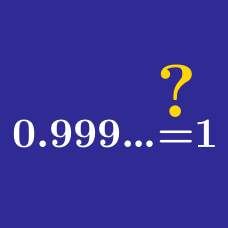Algebra

# Algebra Common Misconceptions: Level 3 Challenges

$\large \sqrt{-2}\times\sqrt{-3}=\, ?$

In this problem, the square root is a function from the complex numbers to the complex numbers.

If $x$ and $y$ are non-zero numbers such that $x>y$, which of the following is always true?

(A) $\dfrac{1}{x}<\dfrac{1}{y}$

(B) $\dfrac{x}{y}>1$

(C) $|x|>|y|$

(D) $\dfrac{1}{xy^2}>\dfrac{1}{x^2y}$

(E) $\dfrac{x}{y}>\dfrac{y}{x}$

Calvin has a collection of special weighted dice that all share special properties:

• They're all 4 sided dice
• Any one die has distinct positive integers on each of its faces
• No pair of dice have all their numbers exactly the same
• The probability of rolling number $x$ on any one of the dice is $\frac {1}{x}$

Let $a$ be the maximum number of dice in the collection and let $S$ be the sum of all the faces of all the dice in the maximum collection size.

Find $a+S$.

I claim that $1 < -1$ using the proof below but I was just told that I might have just committed a small mistake.

A. $- ( i^4 - i^3 - i - 1)< 1 -i^2$
B. $i^3 - i^4 + i + 1 < 1-i^2$
C. $(i^3+i)(1-i) < (1-i)(1+i)$
D. $i^3 + i < 1+i$
E. $i^2(1+i) < 1+i$
F. $i^2 < 1$
G. $i^2 < i^4$
H. $1 < i^2$
I. $1 < -1$

Where did I go wrong? At which step(s) did I commit a fallacy?

Note: $i = \sqrt {-1}$.

What is the wrong step in the following proof that $1 = -1$?

1. Let $w$ be a complex number such that $(w + 1)^3 = (w - 1)^3$.

2. Solving this equation gives $w = \pm \frac{i \sqrt{3}}{3}$.

3. Since $(w + 1)^3 = (w - 1)^3$ for our previously mentioned values of $w$, cube rooting both sides gives $w + 1 = w - 1$.

4. Subtracting $w$ from both sides gives $1 = -1$.

×